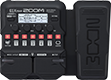# Greenday Patch

Discussion in 'Zoom G1/G1X Four' started by David Mattheus Dee, Jun 14, 2020.

1. Greenday PatchDevice: Zoom G1X Four
Firmware: 1.10

Name on device: Greenday
Optimized for: Phones/Speaker

Effects chain:Dookie Drive

Effect: "ZNR" (Dynamics), active - "yes"
"Detect" = EFXIN
"Depth" = 100
"Threshold" = 11
"Decay" = 0

Effect: "TS Drive" (Overdrive / Distortion), active - "yes"
"Gain" = 98
"Boost" = On
"Tone" = 22
"Volume" = 82

Effect: "MS 800" (Amp simulator), active - "yes"
"Input" = HI
"Bass" = 58
"Middle" = 57
"Treble" = 32
"Presence" = 82
"Gain" = 47
"Volume" = 50

Effect: "DIST Plus" (Overdrive / Distortion), active - "yes"
"Gain" = 60
"Volume" = 84
"DryMx" = 12
"Comp" = ORG

Effect: "Gt GEQ 7" (Filter), active - "yes"
"100" = 12.0
"200" = -0.5
"400" = 0.5
"800" = 0.5
"1.6k" = 3.5
"3.2k" = 2.0
"6.4k" = 0.0
"VOL" = 80

Patch Volume: 100